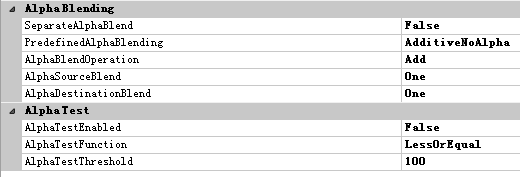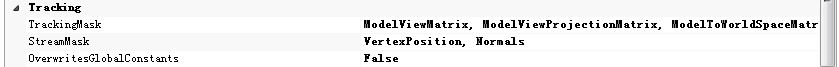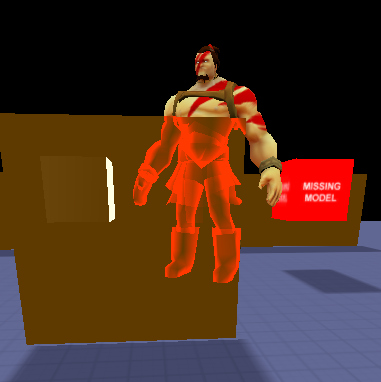# 在Havok Project Anarchy 中创建透视效果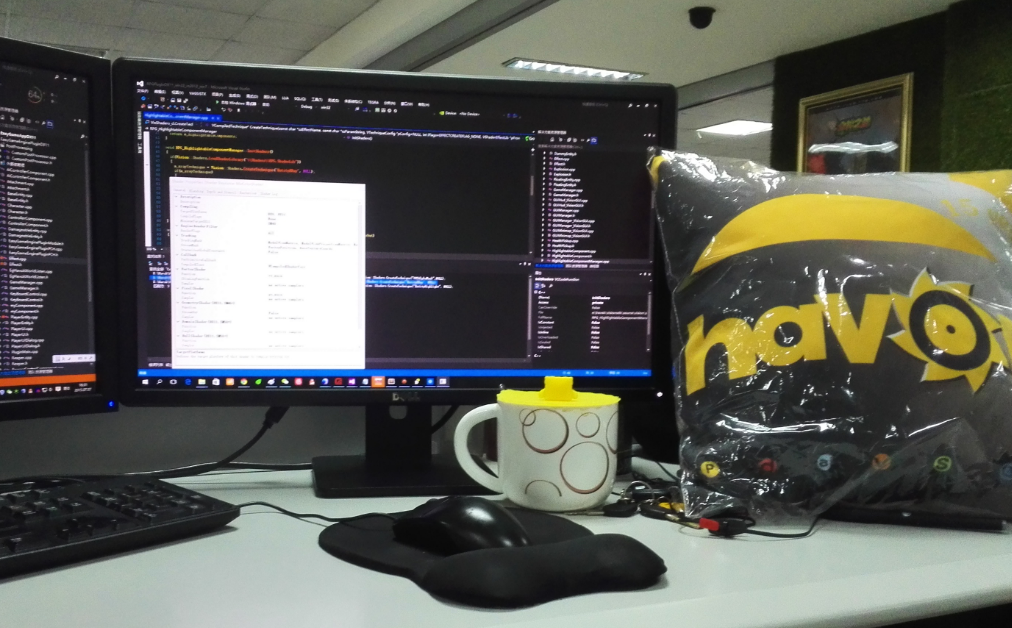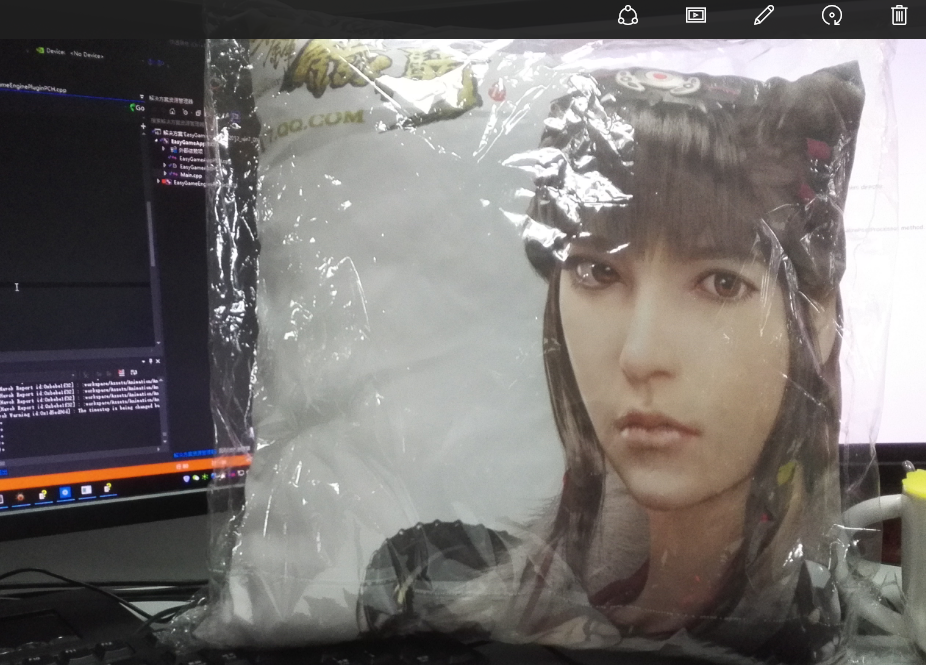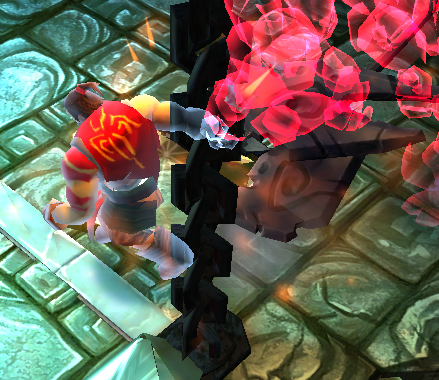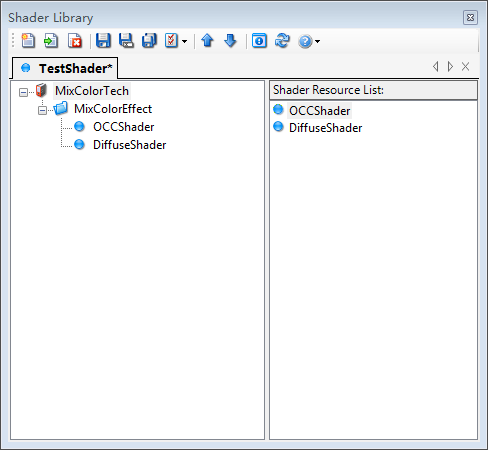```#include <Shaders/VisionCommon.inc>

float4x4 matMV : register(c0);
float4x4 matMVP : register(c8);

#if defined(_VISION_GLES2)
float4 FuckColor : register(c0);
#else
float4 FuckColor;
#endif

struct Test_In
{
float4 VectPos : POSITION;
float3 Normal : NORMAL;
};

struct Test_Out
{
float4 ProjPos : SV_POSITION;
float4 COLOR : COLOR;
};

Test_Out vs_main( Test_In In )
{
Test_Out output;
output.ProjPos = mul(matMVP, In.VectPos);

float3 vNormal = normalize( mul( (float3x3)matMV, In.Normal ));
float3 vVertexDir = -normalize(mul(matMV, In.VectPos).xyz);
float vDir = (1.0 - dot(vNormal, vVertexDir));
output.COLOR = FuckColor;
output.COLOR.a *= vDir;

return output;
}
```

float3 vNormal = normalize( mul( (float3x3)matMV, In.Normal )); //将模型法线转换到世界坐标系下,并归一化（区间 -1 ~ 1)
float3 vVertexDir = -normalize(mul(matMV, In.VectPos).xyz); //将模型顶点转换到世界坐标系下，归一化后成为顶点方向
float vDir = (1.0 – dot(vNormal, vVertexDir)); //点乘顶点和法线的向量，求出它们的夹角，然后用1.0 减去这个夹角，因为都是单位向量，因此减法后刚好可以当作 0~1.0 的透明度值用。

[cc language=”cpp”]
sampler2D BaseTexture : register(s0);

struct Test_In
{
float4 ProjPos : SV_POSITION;
float4 COLOR : COLOR;
};

float4 ps_main( Test_In In ) : SV_Target
{
return In.COLOR;
}
[/cc]

FuckColor 是一个参数，可以在PA中设置它的颜色参数。

DepthTestEnabled : false //让需要透视的物体像素不进行深度检测
DepthWriteEnabled : false //这样位于透视物体前的物体也无法进行像素深度检测
DepthComparisionFunction : Grater //比较条件为深度大于源深度

[cc language=”cpp”]

float4x4 matMV : register(c0);
float4x4 matMVP : register(c8);

struct Test_In
{
float4 VectPos : POSITION;
float4 UV : TEXCOORD0;
};

struct Test_Out
{
float4 ProjPos : SV_POSITION;
float4 UV : TEXCOORD0;
};

Test_Out vs_main( Test_In In )
{
Test_Out output;
output.ProjPos = mul(matMVP, In.VectPos);
output.UV = In.UV;
return output;
}
[/cc]

[cc language=”cpp”]

sampler2D BaseTexture : register(s0);

struct Test_In
{
float4 ProjPos : SV_POSITION;
float4 UV : TEXCOORD0;
};

float4 ps_main( Test_In In ) : SV_Target
{
return vTex2D(BaseTexture, BaseTextureSampler, In.UV);
}
[/cc]